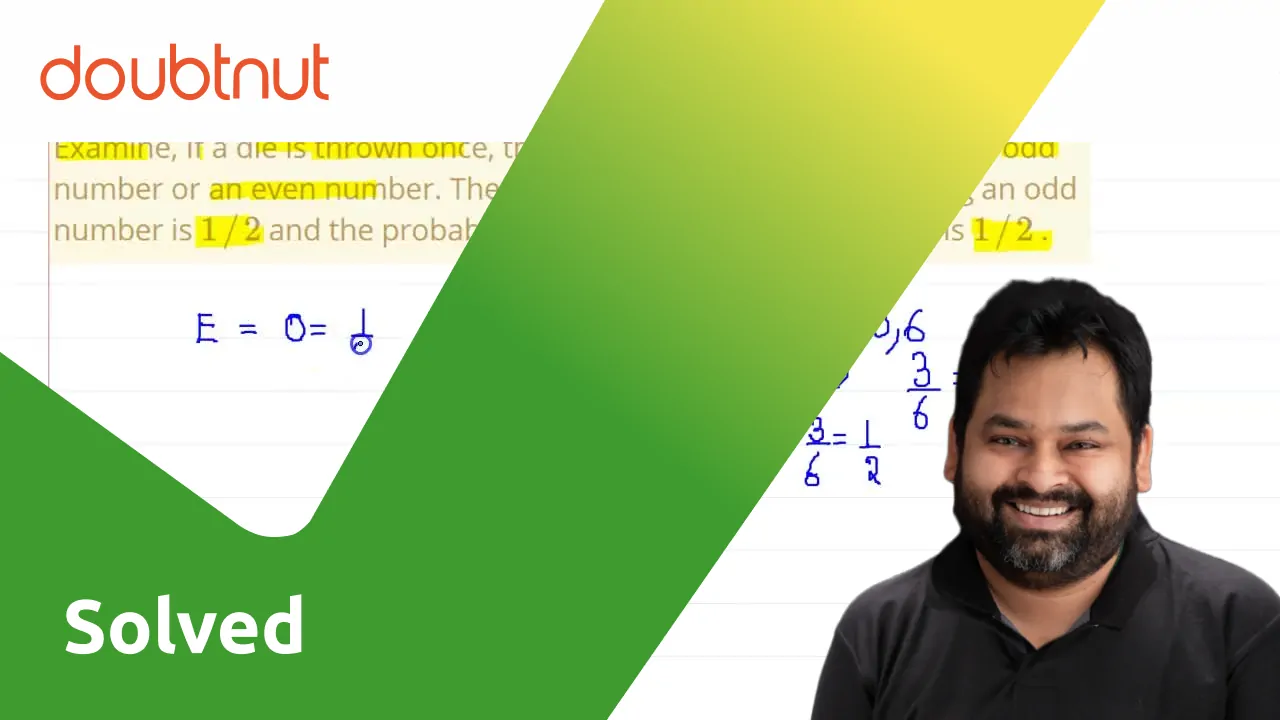Doubtnut App - Get 50 Lakh+ video solutions better than GoogleInstall Now

# Examine, If a die is thrown once, there are two possible outcomes an odd number or an even number. Therefore, the probability of obtaining an odd number is 1/2 and the probability of obtaining an even number is 1/2 .

Video Solution